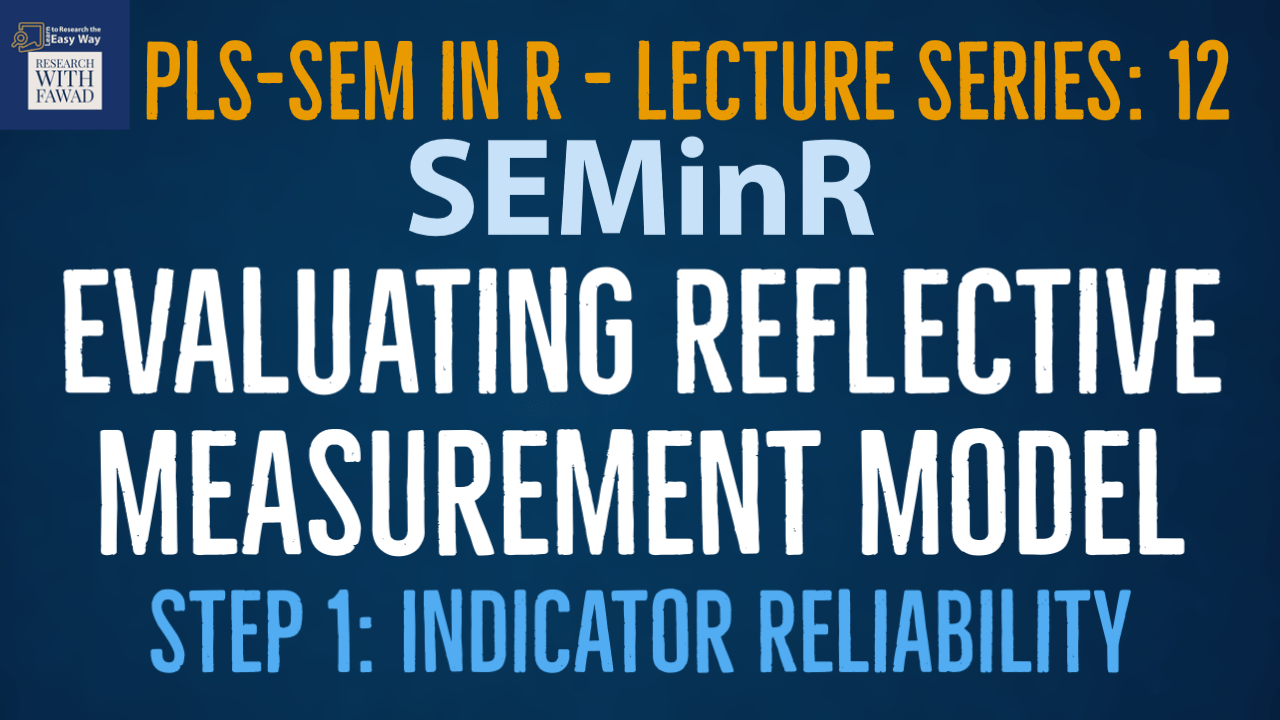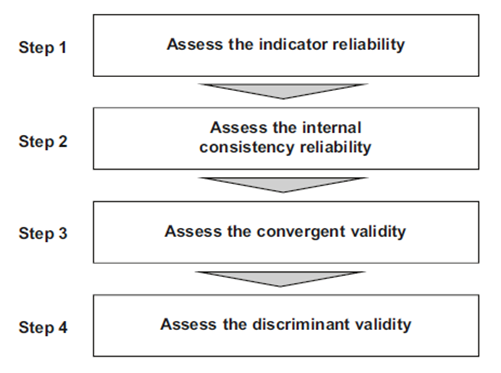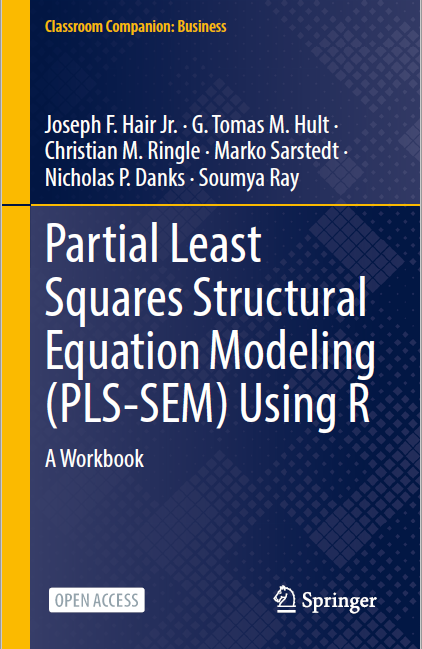# Evaluating Reflective Measurement Model. Step 1: Concept and Indicator Reliability.### SEMinR Lecture Series

This is step 1 of the evaluation of the reflective measurement model. The focus is on the introduction to the reflective measurement model and how to assess indicator reliability.

## Evaluating Reflective Measurement Model

• This section will describe how to evaluate the quality of reflective measurement models estimated by PLS-SEM, both in terms of reliability and validity.
• Assessing reflective measurement models includes evaluating the reliability of measures, on both an indicator level (indicator reliability) and a construct level (internal consistency reliability).
• Validity assessment focuses on each measure’s convergent validity using the average variance extracted (AVE). Moreover, the heterotrait–monotrait (HTMT) ratio of correlations allows assessing a reflectively measured construct’s discriminant validity in comparison with other construct measures in the same model.
• The figure illustrates the reflective measurement model evaluation process.## Specifying Measurement Model

• We continue analyzing the simple PLS path model introduced previously with Vision, Development, and Rewards as Independent and Collaborative Culture as Dependent Variable.
• In the following, we discuss how to evaluate reflective measurement models, using the simple model as an example.
• Recall that to specify and estimate the model, we must first load the data and specify the measurement model and structural model.
• The model is then estimated by using the estimate_pls() command, and the output is assigned to an object.
• In our case study, we name this object simple_model. Once the PLS path model has been estimated, we can access the reports and analysis results by running the summary() function.
• To be able to view different parts of the analysis in greater detail, we suggest assigning the output to a newly created object that we call summary_simple in our example.
``````library(seminr)
# Create measurement model
simple_mm <- constructs(
composite("Vision", multi_items("VIS", 1:4)),
composite("Development", multi_items("DEV", 1:7)),
composite("Rewards", multi_items("RW",1:4)),
composite("Collaborative Culture", multi_items("CC", 1:6)),
composite(“Organizational Performance”, multi_items(“OP”, 1:5)))
# Create structural model
simple_sm <- relationships(
paths(from = c("Vision", "Development", "Rewards"), to = "Collaborative Culture"),
paths(from = “Collaborative Culture”, to = “Organizational Performance”))
# Estimate the model
simple_model <- estimate_pls(data = datas, measurement_model = simple_mm, structural_model = simple_sm)
# Summarize the model results
summary_simple <- summary(simple_model)``````

## Results

• Note that the results are not automatically shown but can be extracted as needed from the summary_simple object.
• For a reminder on what is returned from the summary() function applied to a SEMinR model and stored in the summary_simple object, refer to Table 2 (Bottom).
• Before analyzing the results, we advise to first check if the algorithm converged (i.e., the stop criterion of the algorithm was reached and not the maximum number of iterations – see Table 1 (Upper) for setting these arguments in the estimate_pls() function).
• To do so, it is necessary to inspect the iterations element within the summary_simple object by using the \$ operator.
• This number should be lower than the maximum number of iterations (e.g., 300).
``````#Iterations to converge
summary_simple\$iterations``````
• If the PLS-SEM algorithm does not converge in fewer than 300 iterations, which is the default setting in most PLS-SEM software, the algorithm could not find a stable solution.
• This kind of situation almost never occurs. But if it does occur, there are two possible causes:
• The selected stop criterion is set at a very small level (e.g., 1.0E-10 as opposed to the standard of 1.0E-7), so that small changes in the coefficients of the measurement models prevent the PLS-SEM algorithm from stopping, or
• There are problems with the data, and it needs to be checked carefully.
• For example, data problems may occur if the sample size is too small or if the responses to an indicator include many identical values (i.e., the same data points, which results in insufficient variability, error message is singular matrix).

## Indicator Reliability

• The first step in reflective measurement model assessment involves examining how much of each indicator’s variance is explained by its construct, which is indicative of indicator reliability.

• To compute an indicator’s explained variance, we need to square the indicator loading, which is the bivariate correlation between indicator and construct.

• As such, the indicator reliability indicates the communality of an indicator.

• Indicator loadings above 0.708 are recommended, since they indicate that the construct explains more than 50 percent of the indicator’s variance, thus providing acceptable indicator reliability.

## Assessing Indicator Reliability

• In the following, we inspect the summary_simple object to obtain statistics relevant for assessing the construct measures’ internal consistency reliability, convergent validity, and discriminant validity.
• For the reflective measurement model, we need to estimate the relationships between the reflectively measured constructs and their indicators (i.e., loadings).
• The results for the indicator loadings can be found by using the \$ operator when inspecting the summary_simple object.
• The calculation of indicator reliability can be automated by squaring the values in the indicator loading table by using the ^ operator to square all values (i.e., ^2):
• All indicator loadings of the reflectively measured constructs are well above the threshold value of 0.708 (Hair, Risher, Sarstedt, & Ringle, 2019), which suggests sufficient levels of indicator reliability. Variance is explained by taking Square of the loadings, indicating indicator reliability if its is over .50
``````# Inspect the indicator loadings
# Inspect the indicator reliability

## Improving Indicator Reliability

• Researchers frequently obtain weaker indicator loadings (< 0.708) for their measurement models in social science studies, especially when newly developed scales are used.

• Rather than automatically eliminating indicators when their loading is below 0.70, researchers should carefully examine the effects of indicator removal on other reliability and validity measures.

• Generally, indicators with loadings between 0.40 and 0.708 should be considered for removal only when deleting the indicator leads to an increase in the internal consistency reliability or convergent validity (discussed in the next sections) above the suggested threshold value.

• Another consideration in the decision of whether to delete an indicator is the extent to which its removal affects content validity, which refers to the extent to which a measure represents all facets of a given construct.

• As a consequence, indicators with weaker loadings are sometimes retained. Indicators with very low loadings (below 0.40) should, however, always be eliminated from the measurement model .

## Complete Code

``````library(seminr)
# Create measurement model
#For Multi_Items Identified Separately
#composite("Vision", c("VIS1","VIS2","VIS3","VIS4")
simple_mm <- constructs(
composite("Vision", multi_items("VIS", 1:4)),
composite("Development", multi_items("DEV", 1:7)),
composite("Rewards", multi_items("RW",1:4)),
composite("Collaborative Culture", multi_items("CC", 1:6)),
composite("Organizational Performance", multi_items("OP", 1:5)))
# Create structural model
simple_sm <- relationships(
paths(from = c("Vision","Development","Rewards"), to = "Collaborative Culture"),
paths(from = "Collaborative Culture", to = "Organizational Performance"))
# Estimate the model
simple_model <- estimate_pls(data = datas,
measurement_model = simple_mm,
structural_model = simple_sm)
# Summarize the model results
summary_simple <- summary(simple_model)
#To Display all the contents in the summary_simple Object
summary_simple
# Iterations to converge
summary_simple\$iterations

# Inspect the indicator reliability

# Write the indicator reliability to csv file

## Reference

Hair Jr, J. F., Hult, G. T. M., Ringle, C. M., Sarstedt, M., Danks, N. P., & Ray, S. (2021). Partial Least Squares Structural Equation Modeling (PLS-SEM) Using R: A Workbook.The tutorials on SEMinR are based on the mentioned book. The book is open source and available for download under this link.